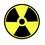# Perfect Cuboid (unsolved)

Given four equations

$a^2+b^2=d^2$

$a^2+c^2=e^2$

$b^2+c^2=f^2$

$a^2+b^2+c^2=g^2$

a,b,c,d,e,f and g are positive integers.

And the problem is to find a triplet (hard) or prove that there are no pairs. (harder)

This problem is calles the perfect cuboid as if a,b and c are the sides of a cuboid, d,e and f are the face diagonnals and g is the space diagonnal. And all of these are integers.

So lets try to solve this?

For a start, I have shown that for integers x, y and z,

$a = 2xz$

$b = 2yz$

$c = x^2+y^2-z^2$

$d = 2z \sqrt{x^2+y^2}$

$e = \sqrt {[(y+z)^2+x^2][(y-z)^2+x^2]}$

$f = \sqrt {[(x+z)^2+y^2][(x-z)^2+y^2]}$

$g = x^2+y^2+z^2$

This is because for $a^2+b^2+c^2=g^2$, a, b, c and g must be in the form given above.

Then, $d = \sqrt{g^2-c^2}, e = \sqrt{g^2-b^2}, f = \sqrt{g^2-a^2}$Note by Aloysius Ng
6 years, 7 months ago

This discussion board is a place to discuss our Daily Challenges and the math and science related to those challenges. Explanations are more than just a solution — they should explain the steps and thinking strategies that you used to obtain the solution. Comments should further the discussion of math and science.

When posting on Brilliant:

• Use the emojis to react to an explanation, whether you're congratulating a job well done , or just really confused .
• Ask specific questions about the challenge or the steps in somebody's explanation. Well-posed questions can add a lot to the discussion, but posting "I don't understand!" doesn't help anyone.
• Try to contribute something new to the discussion, whether it is an extension, generalization or other idea related to the challenge.

MarkdownAppears as
*italics* or _italics_ italics
**bold** or __bold__ bold
- bulleted- list
• bulleted
• list
1. numbered2. list
1. numbered
2. list
Note: you must add a full line of space before and after lists for them to show up correctly
paragraph 1paragraph 2

paragraph 1

paragraph 2

[example link](https://brilliant.org)example link
> This is a quote
This is a quote
    # I indented these lines
# 4 spaces, and now they show
# up as a code block.

print "hello world"
# I indented these lines
# 4 spaces, and now they show
# up as a code block.

print "hello world"
MathAppears as
Remember to wrap math in $$ ... $$ or $ ... $ to ensure proper formatting.
2 \times 3 $2 \times 3$
2^{34} $2^{34}$
a_{i-1} $a_{i-1}$
\frac{2}{3} $\frac{2}{3}$
\sqrt{2} $\sqrt{2}$
\sum_{i=1}^3 $\sum_{i=1}^3$
\sin \theta $\sin \theta$
\boxed{123} $\boxed{123}$

Sort by:

No perfect cuboids have been found with side lengths under $10^{10}$. Not to discourage you, but things like these are unsolved for a reason.

- 6 years, 7 months ago

I know... Thats why im trying some other methods...

- 6 years, 7 months ago

I think it is conjecture that four dimensional Euler Bricks have no solution but a three dimensional Euler Brick has solutions smallest one is(44,117,240),where face diagonals are 125,244,267.This problem not fully similar to Euler Brick as Euler Brick's Diophantine equations was was the first the three equations you written not the fourth one a^2+b^2+c^2=g^2

- 6 years, 7 months ago

Sorry, I confused the names between an Euler Brick and a perfect cuboid.

- 6 years, 7 months ago

Yes it is okay,they are almost similar but not fully similar.

- 6 years, 7 months ago

Also, see that d^2+e^2+f^2=2*g^2. So if a,b,c is a perfect cuboid combination, then it is not possible for d,e,f to also form a perfect cuboid combination. Where d,e,f are the sides of a new cuboid and d^2+e^2+f^2=L^2 such that L is the space diagonal.

- 3 years, 4 months ago